The calculators on this page require JavaScript but you appear to have switched JavaScript off (it is disabled). Please go to the Preferences for this browser and enable it if you want to use the calculators, then Reload this page.
Is there a formula for the n-th Fibonacci number F(n) in terms of only n?
How many digits does F(n) have?
Given one Fibonacci number can we compute the next directly using only that number?
How can I tell if a given number is a Fibonacci number?
All these questions are answered on this page, using no more maths than you meet at school (UK, up to age 16).
The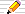icon means there is a Things to do section of questions to start your own investigations. The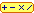calculator icon indicates that there is a live interactive calculator in that section.

0, 1, 1, 2, 3, 5, 8, 13, 21, 34, 55, 89, 144, 233, 377, 610, 987 ..More..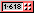## Binet's Formula for the nth Fibonacci number

We have only defined the nth Fibonacci number in terms of the two before it:
the n-th Fibonacci number is the sum of the (n-1)th and the (n-2)th.
So to calculate the 100th Fibonacci number, for instance, we need to compute all the 99 values before it first - quite a task, even with a calculator!
A natural question to ask therefore is:
Can we find a formula for F(n) which involves
only n and does not need any other (earlier) Fibonacci values
?
Yes! It involves our golden section number Phi and its reciprocal phi:
Here it is:
 Fib(n) = Phin − (−Phi)−n = Phin − ( −phi)n √5 √5

On these pages we use
 Phi = √5 + 1 =   1·61803 39887 49894 84820 ... and phi = Phi −1 = 1 = √5 −1 = 0·61803 39887 49894 84820 ... 2 Phi 2
The next version uses just one of the golden section values: Phi, and all the powers are positive:
Fib(n) =
 Phin – (–1)nPhin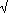5

Since phi is the name we use for 1/Phi on these pages, then we can remove the fraction in the numerator here and make it simpler, giving the second form of the formula at the start of this section.

 We can also write this in terms of5 since Phi = 1 +5 and –phi = 1 –5 :22

 Fib(n) = Phin – (–phi)n = Phin–(–phi)n = 11 + √5n –1 – √5nPhi – (–phi) √5 √5 2 2

If you prefer values in your formulae, then here is another form:-

 Fib(n) = 1.6180339..n – (–0.6180339..)n2.236067977..

This is a surprising formula since it involves square roots and powers of Phi (an irrational number) but it always gives an integer for all (integer) values of n!

Here's how it works: Phi =(1·618..) and -phi=-0·618..: Try some other values of n here, but remember that this calculator has some built-in limits for the number of decimal places it can accurately compute. Sometimes these inaccuracies will make numbers round to the wrong values.
You should be suspicious of large numbers that end with 0 because this could indicate a loss of some of the final digits. Only the left-hand digits of a large number are correct -- the question is just how many!

But the mathematics does tell us that the resulting Fib(n) really should be an integer for all values of n! We will return to this problem in the next section.

###A Calculator for F(n) using Binet's Formula

C A L C U L A T O R
Fib(n) using Binet's Formula with n=
R E S U L T S:: Binet's Formula Approximating F(n)'s Number of Digits Is N a Fibonacci number? Nearest Fibonacci Number ≤ n
You might want to look at two ways to prove this formula: the first way is very simple and the second is more advanced and is for those who are already familiar with matrices.

### An approximation is good enough

Since phi is less than one in size, its powers decrease rapidly. We can use this to derive the following simpler formula for the n-th Fibonacci number F(n):

F(n) = round( Phin / √5 ) provided n ≥ 0
where the round function gives the nearest integer to its argument. Notice how, as n gets larger, the value of Phin/√5 is almost an integer.
If n<0 then the powers of (-phi) become larger than the same power of Phi, so then we use
F(n) = round( (-phi)n / √5 ) provided n < 0

####An Approximating Calculator

C A L C U L A T O R
rounded powers of Phi and (-phi) when n=
R E S U L T S:: Binet's Formula Approximating F(n)'s Number of Digits Is N a Fibonacci number? Nearest Fibonacci Number ≤ n

####Things to do1. What is F(100) according to Binet's formula?
The first calculator will give you some of the initial digits, but the right-hand digits will be wrong. You may choose to write a computer program for this, or use a package (such as Mathematica or Maple) which lets you work out very long integers exactly.
2. How many digits does F(100) have? (the approximate value on the first calculator above should tell you). Check your answer with the list of Fibonacci numbers.
3. Look at the following table:
n: Phin=X: (-Phi)-n=Y: X-Y: (X-Y)/(5):
11·618033989 -0·61803399 2·23606798 1
You might nave noticed that we didn't ADD the X and Y values to get 1·618..-0·618..=1 directly but instead in Binet's Formula, we subtracted and divided by sqrt(5).
Let's see what happens if we do just ADD the X and Y columns:
(a) Add a new column to the table above which is X+Y. Fill it in and you'll notice something very surprising - another integer series that is not the Fibonacci numbers!! These numbers are called the Lucas Numbers and they also have some similar properties to the Fibonacci numbers and are covered in another page at this site (see Fibonacci Home page).
(b) Can you spot the rule whereby the latest two Lucas numbers are used to generate the next Lucas number?

### Historical Note - Binet's, de Moivre's or Euler's Formula?

Many authors say that this formula was discovered by J. P. M. Binet (1786-1856) in 1843 and so call it Binet's Formula.
Graham, Knuth and Patashnik in Concrete Mathematics (2nd edition, 1994) mention that Euler had already published this formula in 1765.
But Don Knuth in The Art of Computer Programming, Volume 1 Fundamental Algorithms, section 1.2.8, traces it back even further, to A de Moivre (1667-1754). He had written about "Binet's" formula in 1730 and had indeed found a method for finding formulae for any general series of numbers formed in a similar way to the Fibonacci series.
Like many results in Mathematics, it is often not the original discoverer who gets the glory of having their name attached to the result, but someone later!

0, 1, 1, 2, 3, 5, 8, 13, 21, 34, 55, 89, 144, 233, 377, 610, 987 ..More..## How many digits does a number have?

### Using the display on your calculator

One of the questions above asks you to use your calculator to find out how many digits are in a number. When the number gets too big for the calculator's display, it shows the first few digits and a little "exponent" which says how to move the decimal place from where it is shown to it true place - negative means move it to the left, otherwise move it to the right from where it is shown in the display.
So Phi20/sqrt(5) on my calculator is 6765·000029 and Fib(20)=6765.
But Phi60/sqrt(5) shows as 1·548008755 12 where the little figures at end are the "exponent", that is, the true value is 1·548008755x1012. If we move the decimal point 12 places to the right (putting in 0s for the missing digits), we get:
1548008755000. and the correct value for Fib(60) is
1548008755920
So the exponent, when positive, has told us how many digits there are in the number calculated, showing just the first few of the digits if not all of them will fit into the display window!

Similarly, phi60 is just 1/Phi60 which we've just calculated. Using the "1/x" button on my calculator when it is showing the value above gives: 6·459911784-13 meaning 6·459911784x10-13. This time we must move the decimal place to the left since the exponent is negative and we must move it 13 places. This gives 0·00000000000064511784 as the value for phi60 - quite small!

### Using the LOG button on your calculator

But how can we calculate the number of digits in a given whole number?
This section shows how to use the LOG button on your calculator to find out how long a number is.

Returning to the investigation above where you calculated F(100), this number is usually too big for most calculators to compute, but we can find how long it is as follows, using the simplified formula:

Fib(n) = round( Phin/√5 )
[This very nearly gives the correct value of F(n) since the part of the formula we have omitted is very small indeed for large n.]
The LOG button on your calculator can be used to compute how long a number is, that is, how many decimal digits it has.
• This is the "logarithm to base 10". Another button, usually labelled LN is the "logarithm to base e".
• Take the LOG of any 3-digit number and the answer should be "2 point something".
Try with any 4-digit number and you get a LOG of "3.something". So,
the number of digits in any integer is just 1+ the whole-part of its LOG.
• LOGs have useful properties such as:
• if we ADD LOGS we find the length of the PRODUCT of the original numbers;
e.g. the LOG of x2 is just 2 times LOG x and
the LOG of x3 = 3 LOG x
• if we SUBTRACT LOGS we find the length of the QUOTIENT (DIVISION):
e.g. the LOG of √x = (LOG x)/2

### How many digits are there in Fib(n)?

So, now you have enough information to answer the question:
How many digits has F(1000)?
Computing LOG (Phi1000/√5) is the same as computing
1000*LOG(Phi) - (LOG √5) = 1000*LOG Phi - (LOG 5)/2 = 208.638155.
So 1+the whole number part of your answer is the number of digits in F(1000), i.e. 209 digits.
In fact, you can find the first few digits by using the rest of the LOG answer as we'll see in the next section.

### What are the first few digits of Fib(n)?

The whole number part of the log tells us how many digits are in the number. The rest of the log of a number tells us what those initial digits are. This is because if the log of the number is xxxx.yyyyyy then the number itself is 10xxxx.yyyyyy=10xxxx+0.yyyyyy=10xxxx x 100.yyyyyy
10xxxx is just a power of ten so it tells us how to move the decimal point in 100.yyyyyy.
Since 0.yyyy is between 0 and 1 and 100=1 and 101=10 then
100.yyyyyy has a single non-zero digit before its decimal point.

The part after the decimal point in number r is often called the fractional part of r and written as frac(r).
So the first few digits of a number r are given by 10frac(log10(r)).

####Number of Digits in F(n) Calculator

C A L C U L A T O R
 i= up to

R E S U L T S: Binet's Formula Approximating F(n)'s Number of Digits Is N a Fibonacci number? Nearest Fibonacci Number ≤ n

## Finding Fibonacci Numbers Fully

If we want to study the full and exact integer form of a Fibonacci number, how do we do it? We will assume that our calculator or computer can add, subtract and multiply whole numbers of any length (such as Maple or Mathematica). [If not, it is an interseting and easy programming exercise to represent integers of any length as an array of single digits together with functions that manipluate them to perform addition, subtraction and multiplication using the rules that we all learned at school.] We have the basic recursive definition, of course (where recursive just means that we are defining one value of F in terms of two other, simpler, values of F):
F(0)=0
F(1)=1
F(n) = F(n-1) + F(n-2), n>1
but the disadvantage is that to calculate F(1000), for example, we need to compute all 999 numbers before it.
Alternatively, using Binet's formula above means we need to compute many decimal places of √5.
So the question we have is:
Can we find a quicker method using only integers?
One method was proposed by the late Prof. Edsgar W Dijkstra around 1978, described in note EWD654 "In honor of Fibonacci" (PDF, 38K; download the free Acrobat Reader to view it if your browser will not display it).
Note that Dijkstra's series begins F(1)=0 and F(2)=1 so, using our index system which has F(0)=0 and F(1)=1, we have:
F(2n-1) = F(n-1)2 + F(n)2
F(2n) = ( 2 F(n-1) + F(n) ) F(n)
which need only F(n) and F(n-1) to compute both F(2n) and F(2n-1). Although the formula in Dijkstra's note were "well-known" in 1978, his method of using these two formula in this way as an efficient algorithm for computing big Fibonacci numbers may be original.
So, to compute F(1000) we would use the two formulae as follows:
• F(1000) needs F(500) and F(499)
• F(500) and
F(499) need F(250) and F(249)
• F(250) and
F(249) need F(124) and F(125)
• F(124) needs F(61) and F(62)
• F(125) needs F(62) and F(63)
• F(63) needs F(32) and F(31)
• F(62) and
F(61) needs F(31) and F(30)
• F(32) and
F(31) need F(16) and F(15)
• F(30) needs F(15) and F(14)
• F(16) and
F(15) need F(8) and F(7)
• F(14) needs F(7) and F(6)
• F(8) and
F(7) need F(4) and F(3)
• F(6) needs F(3) and F(2)
• F(4) and
F(3) need F(2) and F(1)
• F(2) needs F(1) and F(0)
• F(1) and
F(0) are 1 and 0 by definition
So there are very few (22) F values needed to compute F(1000) and it takes much less work than using the method of "add the previous two F values to get the next one".

See also Computers use the Rabbit Sequence on the Golden String page at this site for another insight into computers and computing Fibonacci numbers.

0, 1, 1, 2, 3, 5, 8, 13, 21, 34, 55, 89, 144, 233, 377, 610, 987 ..More..## Calculating the next Fibonacci number directly

Suppose we have evaluated Fib(100) and we want to know the next value: Fib(101). Do we have to use Binet's formula again? Well we could do, of course, but here is a short-cut.

There is also a formula that, given one Fibonacci number, returns the next Fibonacci number directly, calculating it in terms only of the previous value (ie not needing the value before as well).

F(n+1) = round( F(n) Phi )
The round function applies to a number (whole or decimal) and changes it to an integer.
• round(x) means "the integer nearest to x".
• If we apply it to a number ending with ·5 then we will round up
eg round(3.5) is 4.
• If we apply the round function to a value which is already a whole number then it does not change it.

### An example

Here's an example of our "next Fibonacci" formula using a small value of n:
Since F(4)=3 then
F(5)
= round( 3 Phi )
= round( 3x1·618... )
= round( 4·854... )
= 5 which is correct!

### But there's a problem....

F(1)=1 and the next Fibonacci number is F(2)=1.
ALSO, F(2)=1 but the next Fibonacci number is F(3)=2.
But we cannot have two different values for "the next Fibonacci number after 1"!
If we put F(n)=1 in the formula, we have round(1 x Phi), or the nearest integer to Phi = 1.618..., which is 2.
So we cannot apply this formula when n is 1 (and you can check that it also fails if n is 0).
So we have an important restriction on the formula above: the formula only applies when n is bigger than 1.

 F(n+1) = round( F(n) Phi )    for all n > 1

### Proving that this formula is correct

You can easily evaluate F(2) from F(1)=1 and F(3) from F(2)=2 by this formula and see that they give F(2)=3 and F(3)=5. Then, if you are familiar with proof by induction you can show that, supposing the formula is true for F(n-1) and F(n) then it must also be true for F(n+1) by showing that adding the formula's expressions for F(n) and F(n-1) gives the formula's expression for F(n+1).

Other ways of proving it involve results about recurrence relations and how to solve them, which are very like solving differential equations, except that they deal with integer values not real number values. This is often included in university level courses on Pure or Discrete Mathematics.

[ For the university level mathematician, there is an interesting HAKMEM note on a fast way of computing Fibonacci numbers and its applications.]

## Detecting when N is a Fibonacci Number

Given a number N it is not easy to spot if it is a Fibonacci number or not.
Is there a simple test to see if N is a Fibonacci number?
I Gessel solved this in 1972 with a surprisingly simple test.
N is a Fibonacci number if and only if 5 N2 + 4 or 5 N2 – 4 is a square number.
For instance,
• 3 is a Fibonacci number since 5x32+4 is 49 which is 72
• 5 is a Fibonacci number since 5x52–4 is 121 which is 112
• 4 is not a Fibonacci number since neither 5x42+4=84 nor 5x42–4=76 are pefect squares.
It is easy to test if a whole number is square on a calculator by taking its square root and checking that it has nothing after the decimal point.
In a computer programming language, take the square root, round it to the nearest integer and then square the result. If this is the same as the original whole number then the original was a perfect square.

###Is N a Fibonacci Number Calculator

C A L C U L A T O R
if is a Fibonacci number
R E S U L T S: Binet's Formula Approximating F(n)'s Number of Digits Is N a Fibonacci number? Nearest Fibonacci Number ≤ n
• Problem H-187: n is a Fibonacci number if and only if 5n2+4 or 5n2-4 is a square posed and solved by I Gessel in Fibonacci Quarterly (1972) vol 10, page 417.
The method above needs to square the number n being tested and then has to check the new number 5 n2 ± 4 is a square number.
If n is large, this can be a problem as n2 has twice as many digits as n.
Another approach is to find the index number i of n or, to put it another way, to find the (index number of the) closest number equal to or less than n that is a Fibonacci number: so that F(i)≤n but no larger i will have this property.
This involves using logs but can cope with larger numbers that the approach of this current section because the log to base 10 of a number is "the number of digits in a number" even when it is not an exact power of 10. We examine it in the next section.

0, 1, 1, 2, 3, 5, 8, 13, 21, 34, 55, 89, 144, 233, 377, 610, 987 ..More..## Finding the Fibonacci index number i for a given n

It is sometime useful to find the index number i in Fib(i)=n for a given value of n, or to find which index number is close.
Here again Binet's Formula comes in handy - we met it above
 Fib(i) = Phii − ( −phi)i √5
But since phi=0.618 and phi2= 0.382, the powers of phi quickly get very small and have a smaller and smaller effect on Phii. By ignoring the small term we find a simpler formula for Fib(i) from which we can find a formula for the index number i:
 Fib(i) ≈ Phii √5
so that
√5 Fib(i) ≈  Phii
Taking logs of both sides gives:
log(Fib(i) √5) ≈ log( Phii)
and using the log rule that log(a b)=log(a)+log(b) we have:
log(Fib(i)) + log(√5) ≈ log( Phii)
Also we have √a = a1/2 and log(ab) ≈ b log(a) and applying these rules gives:
log(Fib(i)) + log(5)/2 ≈ i log(Phi)
Rearranging we have our formula for the index number i in terms of Fib(i):
 i ≈ log(Fib(i)) + log(5)/2 log(Phi)
If we are given a number N and we wish to find the nearest Fibonacci number below or equal to it, then we compute
 i ≈ log( N ) + log(5)/2 log(Phi)
If N is not an actual Fibonacci number then this value for i will be a fractional value. If we round it down to the nearest whole number then we will have the Fibonacci index-number i where Fib(i) is below or equal to N; i.e. the largest value of i for which Fib(i) ≤ N.
In fact, using either Round(i) (the nearest integer to i) or Floor(i) (the nearest integer below i) on the computed value of i will sometimes result in an error for small values of N which are just below or equal to a Fibonacci number because in these cases the −(−phi)i term that we ignored actually does affect a few results when N is small.
In practice we can use Floor(i) (round i down to the nearest integer) and then test this value and also test (i + 1) to be sure.
In the following Calculator, we indicate where a rounding UP has been necessary in the Results.
We can also precompute log(5)/2 and log(Phi) using logs to base 10 or base e so long as you use the same base for all the logs in the formula. With logs to base 10 to 30 dps we have:
 log(√5) = log(5)/2 = 0.80471 89562 17050 18730 03796 66613 ... log(Phi) = log((√5+1)/2) = 0.48121 18250 59603 44749 77589 13424 ...

###Nearest Fibonacci Number ≤ n Calculator

C A L C U L A T O R : Nearest Fibonacci number ≤ n
the nearest Fibonacci index number i where Fib(i) ≤

R E S U L T S: Binet's Formula Approximating F(n)'s Number of Digits Is N a Fibonacci number? Nearest Fibonacci Number ≤ n

## Binet's Formula for negative n?

Earlier on this page we looked at Binet's formula for the Fibonacci numbers:
Fib(n) = { Phi n - (-phi) n } / √5
Here Phi=1·6180339... and phi = 1/Phi = Phi-1 = (√5-1)/2 = 0·6180339... .

We only used this formula for positive whole values of n and found - surprisingly - it only gives integer results. Well perhaps it was not so surprising really since the formula is supposed to be define the Fibonacci numbers which are integers; but it is surprising in that this formula involves the square root of 5, Phi and phi which are all irrational numbers i.e. cannot be expressed exactly as the ratio of two whole numbers.

Suppose we try negative whole numbers for n in Binet's formula.
The formula extends the definition of the Fibonacci numbers F(n) to negative n.
In fact, if we try to extend the Fibonacci series backwards, still keeping to the rule that a Fibonacci number is the sum of the two numbers on its LEFT, we get the following:

 n : Fib(n): ... -6 -5 -4 -3 -2 -1 0 1 2 3 4 5 6 ... ... -8 5 -3 2 -1 1 0 1 1 2 3 5 8 ...
and this is consistent with Binet's formula for negative whole values of n.
So we can think of Fib(n) being defined an all integer values of n, both positive and negative and the Fibonacci series extending infinitely far in both the positive and negative directions.

## Binet's formula for non-integer values of n?

This section is optional and at an advanced level i.e. post 16 years education.
or learn about square roots of negative numbers in what follows!

Well now we've tried negative values for n, why not try fractional or other non-whole values for n?
This doesn't make sense in terms of numbers in a series (there is a 2nd and a 3rd term and even perhaps a -2nd term but what can we possibly mean by a 2·5th term for instance)??
However, this could give us some interesting (mathematical) insights into the whole-number terms which are our familiar Fibonacci series.

### Complex Numbers

The trouble is that in Binet's formula:

Fib(n) = { Phi n - (-phi) n }/√5
the second term (-phi)n means we have to find the n-th power of a negative number: -phi and n is not a whole number. If n was 0·5 for instance, meaning sqrt(-phi), then we are taking the square-root of a negative value which is "impossible".

Mathematicians have already extended the real-number system to cover such "imaginary" numbers. They are called complex numbers and have two parts A and B, both normal real numbers: a real part, A, and an imaginary part, B. The imaginary part is a multiple of the basic "imaginary" quantity √-1, denoted i. So complex numbers are written as x + i y or x + y i or sometimes as x + I y or even more simply as (x,y).

### Applications of Complex numbers

To me it is still surprising that such "imaginary" numbers - or numbers involving the imaginary quantity that is the square root of a negative number - have very practical applications in the real world.
For instance, electrical engineers have already found many applications for such "imaginary" or complex numbers. Whereas resistance can be described by a real number often measured in ohms, complex numbers are used for the inductance and capacitance, so they have very practical uses!
Electrical engineers tend to use j rather than i when writing complex numbers.

Mathematicians find uses for complex numbers in solving equations:

• Every equation of the form Ax+B=0 has a solution which is a fraction: namely X=-B/A if A and B are integers. These are called linear equations where A and B are, in general, any real numbers.
• Every equation of the form Ax2 + Bx + C=0 has either one or two solutions IF we allow complex numbers for x. (Here A is not zero or we just get a linear equation.)
For instance x2=2 has two solutions:
+sqrt(2) and -sqrt(2)
but x2=0 has just one solution namely x=0.
Note that x2=-2 has two solutions too:
x=sqrt(-2)=isqrt(2) and x=-sqrt(-2)=-isqrt(2)
• Every equation of the form Ax3 + Bx2 + Cx + D = 0 has at most 3 solutions again allowing x to be a complex number if necessary.
This leads to a beautiful theorem about solving equations which are sums of (real number multiples of) powers of x, called polynomials in x:
If the highest power of x in a polynomial is n then there are at most n different complex or real number solutions which make the polynomial's value zero

• Complex Numbers and Their Applications by F J Budden, Longman's 1968,
is now out of print but is an excellent introduction to the fascinating subject of complex numbers and their applications.

### Argand Diagrams

Writing (x,y) for a complex numbers suggests we might be able to plot complex numbers on a graph, the x distance being the real part of a complex number and the y height being its complex part.

Such plots are called Argand diagrams after J. R. Argand (1768-1822).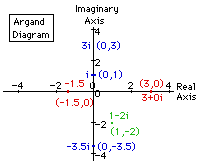We can plot an individual point such as 1 - 2i as the point (1,-2). Numbers which are real have zero as their complex part so the real number 3 is the same as the complex number 3 + 0 i and has "coordinates" (3,0). The real number -1·5 is the same as -1·5 + 0 i or (-1·5,0).
In general, the real number r is the complex number r + 0 i and is plotted at (r,0) on the Argand diagram.
In fact, all the real values are already in the graph along the x axis also called the real axis.
Numbers which are purely imaginary have a real-part of zero and so are of the form 0+yi always lying exactly on the y axis ( the imaginary axis).

### Plotting functions on an Argand Diagram

We can plot a complex function on an Argand diagram, that is, a function whose values are complex numbers. This is where Binet's formula comes in since it will give us complex numbers as n varies over the real numbers.

So what happens if we plot a graph of F(n) described by Binet's formula, plotting the results on an Argand diagram?

The blue plot is for positive values of n from 0 to 6. Note how this curve crosses the x axis (representing the "real part of the complex number") at the Fibonacci numbers, 0, 1, 2, 3, 5 and 8. But there is a loop so it crosses the axis twice at x=1, and we really do get the whole Fibonacci sequence 0,1,1,2,3,5,8.. as the crossing points.
The red plot is of negative values of n from -6 to 0. It also crosses the x axis at the values -8, 5, -3, 2, -1, 1 and 0 corresponding to the Fibonacci numbers F(-6), F(-5), F(-4), F(-3), F(-2), F(-1) and F(0).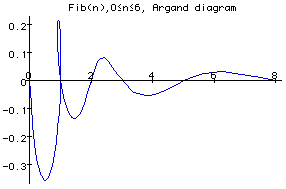Spirals?

• Note that the red spiral for negative values of n is NOT an equiangular or logarithmic spiral that we found in sea-shells on the Fibonacci in Nature page. This is because the curve crosses the x axis at 1 and next at 2, so the distance from the origin has doubled, but the next crossing is not at 4 (which would mean another doubling as required for a logarithmic spiral) but at 5.
• If you adjust the width of your browser window, you should be able to see both curves side by side. Now it looks as if the two curves are made from the same 3-dimensional spiral spring-shape, a bit like the spiral bed-springs in cartoons, getting narrower towards one end. The red curve seems to be looking down the centre of the three-dimensional spring and the blue one looking at the same spring shape but from the side. Comparing the two diagrams we can see that even the heights of the loops are the same!
I haven't yet found an explanation for this - can you find one? [Let me know if you do!]
The plots were produced using parametric plotting function with Maple's built-in "plot" function:
```      Phi:=(sqrt(5)+1)/2;phi:=(sqrt(5)-1)/2;
f:=n->(Phi^n-(-phi)^n)/sqrt(5);
plot([Re(f(n)),Im(f(n)),n=-6..0],color=RED,
title=`Fib(n),-6≤n≤0, Argand diagram`);
plot([Re(f(n)),Im(f(n)),n=0..6],color=BLUE,
title=`Fib(n),0≤n≤6, Argand diagram`);
```
Kurt Papke has a Web page with a Java applet to show the two Argand diagrams but animating the formula that f(n)=f(n-1)+f(n-2) for any real value n. The complex numbers f(n), f(n-1) and f(n-2) can be illustrated as vectors, and so the formula f(n)=f(n-1)+f(n-2) becomes a vector equation showing that the vector f(n-1) added to (followed by) the vector f(n-2) gives the same length-and-direction-movement as the vector f(n).
Kurt has an excellent 3D version of the spiral that you can rotate on the screen (using a Java applet) AND one also for the Lucas numbers formula!

For a different complex function based on Binet's formula, see the following two articles where they both introduce the factor ei nwhich is 1 when n is an integer:

• Argand Diagrams of Extended Fibonacci and Lucas Numbers, F J Wunderlich, D E Shaw, M J Hones Fibonacci Quarterly, vol 12 (1974), pages 233 - 234;
• An Extension of Fibonacci's Sequence P J deBruijn, Fibonacci Quarterly vol 12 (1974) pages 251-258.

### References on Complex Numbers

Complex Numbers are included in some (UK based) Mathematics syllabuses at Advanced level (school/college examinations taken at about age 17). Here are some books relating to different Advanced level Examination Boards syllabus entries on Complex Numbers:© 1996-2016 Dr Ron Knottupdated 18 July 2016